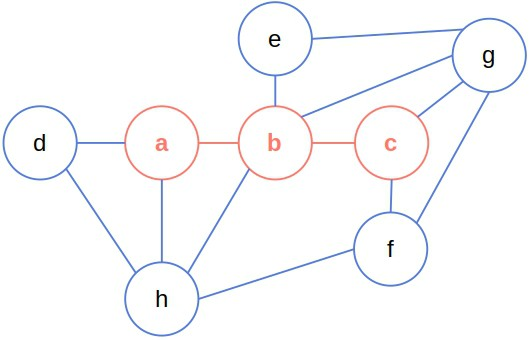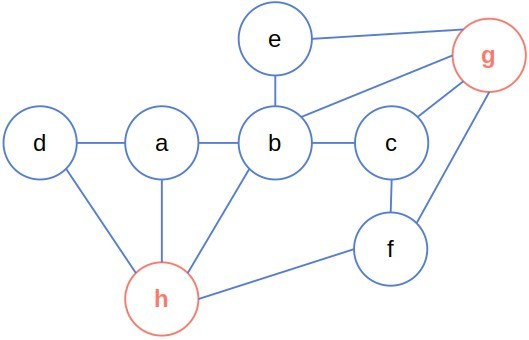## 1. Introduction

In this tutorial, we’ll explain dominating sets and domination numbers of graphs.

## 2. Dominating Sets

A dominating setof the graphis any subset ofsuch that any node out ofis adjacent to at least one node from it.

Formally,is a dominating set ofif:For example,is a dominating set of the following graph:However,is also a dominating set of the previous graph:So, a graph can have multiple dominating sets.

## 3. Domination Number

The domination numberof a graphis the cardinality of its smallest dominating set:Sinceis a minimum dominating set of the previous graph, its domination number is 2.

Checking if the domination number of an arbitrary graphis lower than a given integer is an NP-complete problem. No algorithm is known to do this for all graphs in polynomial time. Still, there are efficient algorithms for approximating the domination numbers and fast methods tailored to particular types of graphs.

## 4. Variations and Applications

We get a connected dominating set if there’s a path between any two nodes inthat goes only through the. So, for example, the first dominating set we previously discussed is connected, but the second one isn’t.

If we require that all the nodes have at least one neighbor in, we get a total dominating set, also known as strongly dominating. The difference between dominating and strongly dominating sets is that the latter requires the dominating nodes to also have at least one dominating neighbor. In contrast, the former requires only this of only the non-dominating nodes.

Dominating sets have many applications. For instance, we use them in routing protocols for ad hoc wireless networks. The idea is to find a dominating set in a network of devices and use the dominating nodes to route the user messages. So, when user A wants to send a message to user B, routing consists of finding the shortest path between the dominating neighbors of A and B. Since all the devices have at least one dominating neighbor, this method guarantees the delivery of messages.

## 5. Conclusion

In this article, we covered the dominating sets and numbers of graphs. Each node out of a graph’s dominating set has at least one dominating neighbor. The domination number of a graph is the cardinality of a minimal dominating set of the graph.